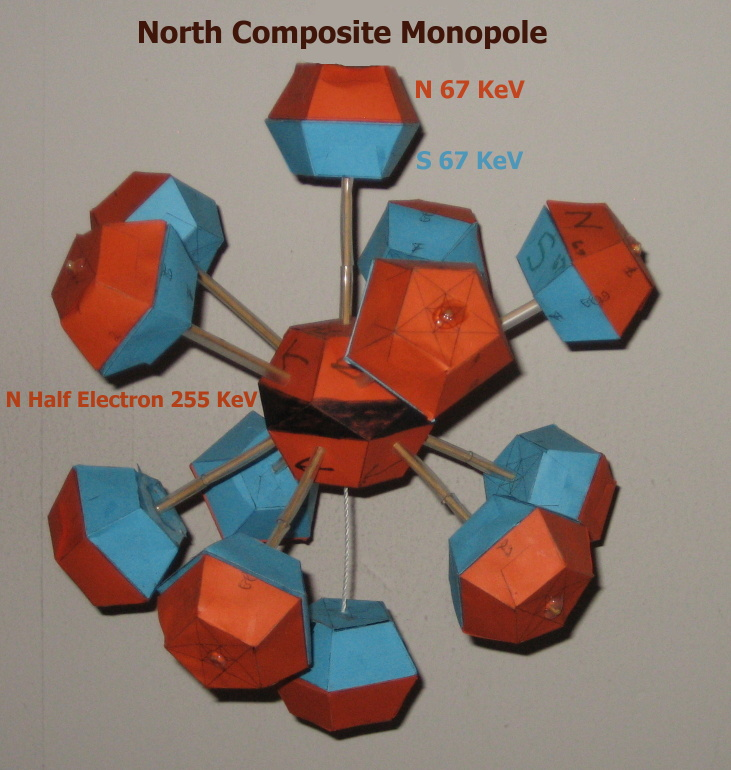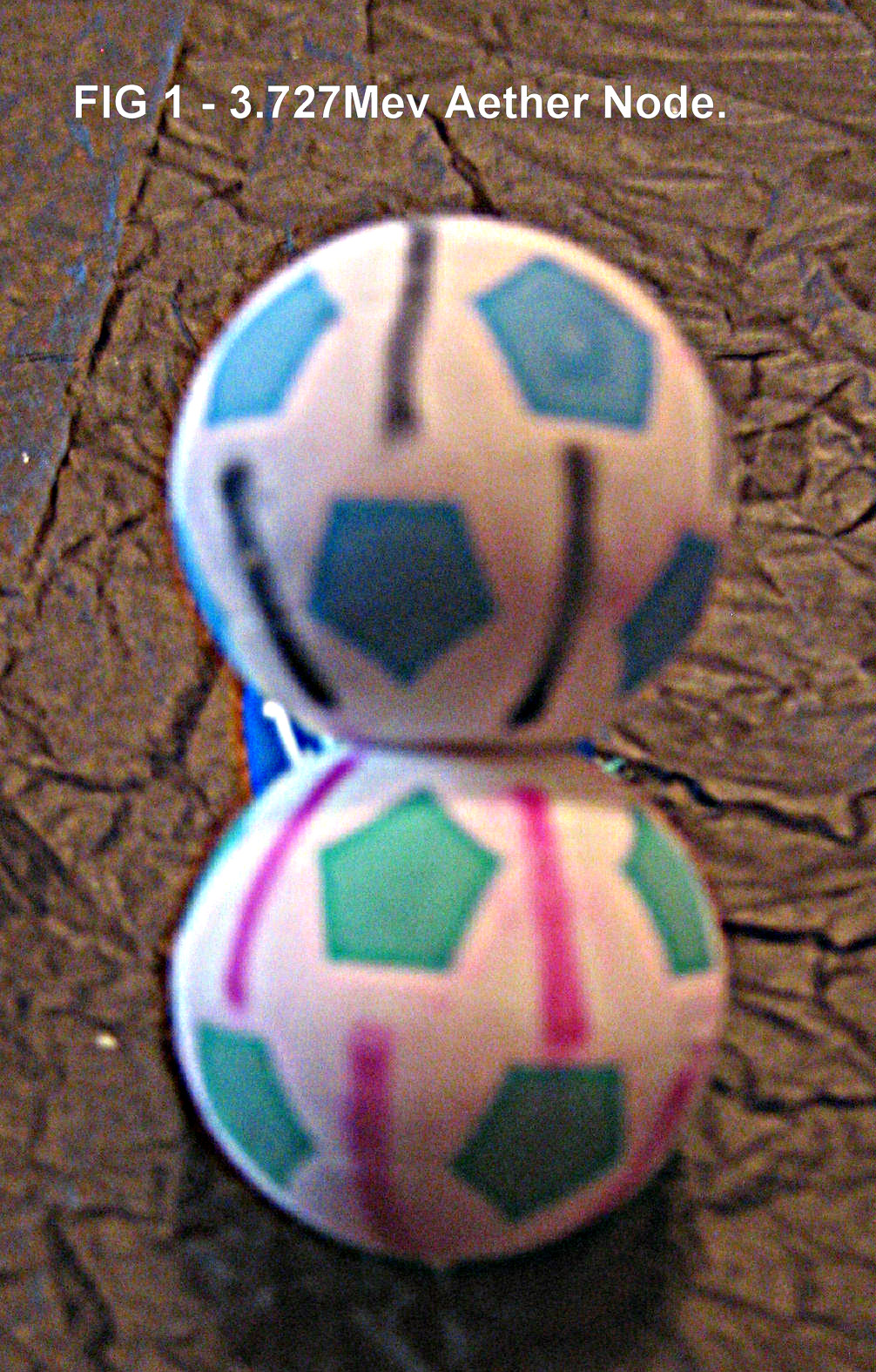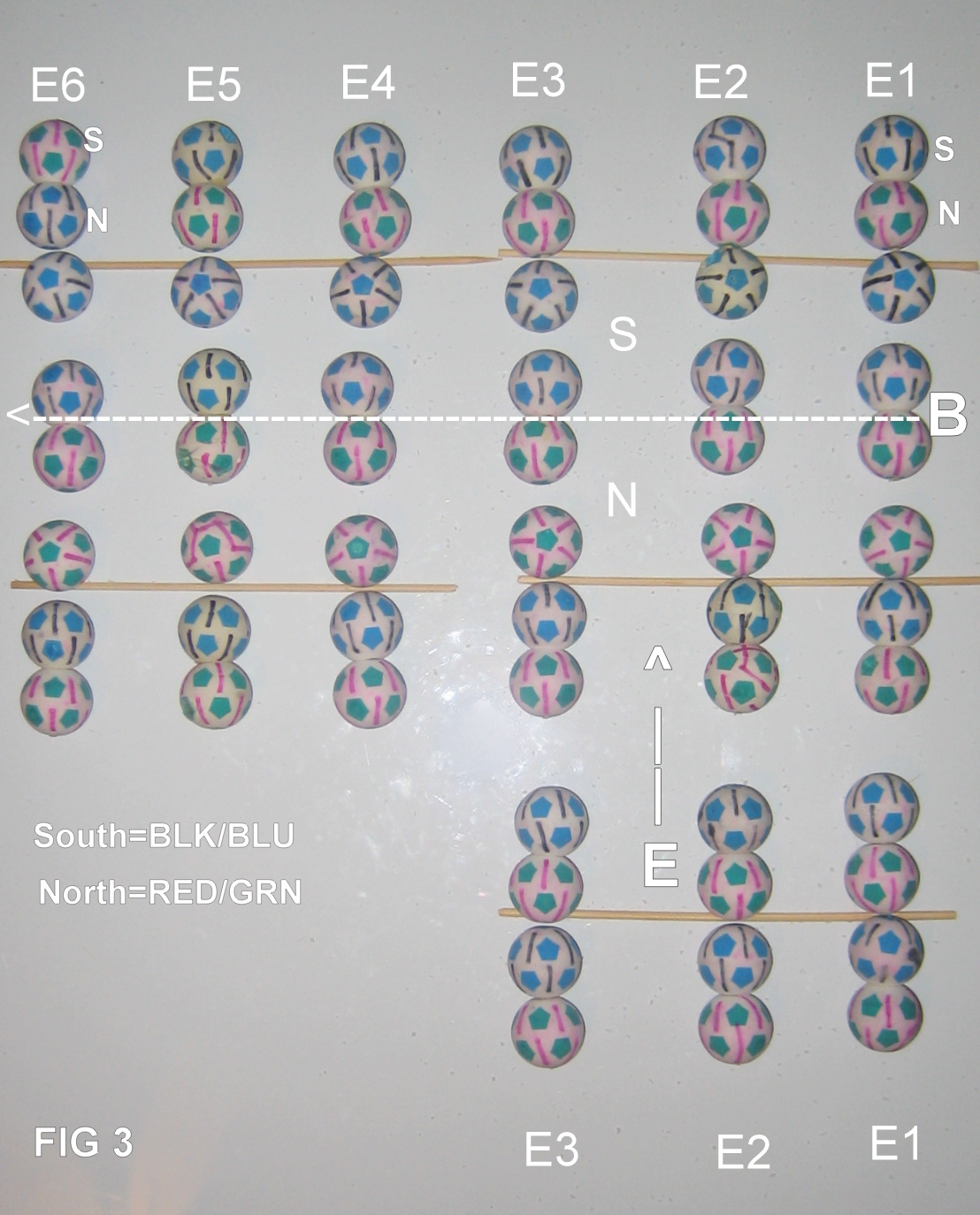NOTICE: THIS MATERIAL IS OBSOLETE [ June 2016 ]

It has been replaced by a draft copy of SOVol: The Structure Of Space as Volume.This paper will be eventually removed as the new material replaces all of it.

## THE Static Monopole Aether Model

Abstract: A 3727 Kev Aether Node model is formed using pairs of 67 Kev and 255 Kev Monopoles.

The model offers possible explanations for the Universe's most fundamental properties,
such as some of the missing mass of the Universe, Pair Production, and the energy density of "empty space".

The model explains Fermion SPIN and all Inertia as caused by
two Monopoles revolving around a common axis.

Gravity and B^3 [Before the Big Bang] models are the major unknowns at present.

### Introduction

Historical experiments have established, if an Aether exists, that it has a relative frame,
and its motion can not be measured .

Once an ubiquitous aether exists, the energy of "empty space" must then reflect
whatever the aether's energy is; as well as its distribution.

All the various current non-Aether models differ in their estimates of this vacuum energy.
In this regard, observed values of the vacuum energy disagree with GR,
as well as with several models; ie QM, Higgs and QCD.

-------------------------------

The mass node at N=3 predicted by the 5SPACE Mass model,
based on stable particle mass nodes at (N/2)^5 ; was used to model various configurations.

Investigation of this 3.88 Mev node revealed resonances at 121 Kev and 134 Kev, based on Inverse Cherenkov experiments.

These two values are the same found in the decay of Cobalt-57 to Iron-57,
in the original experiments of Mossbauer spectroscopy.

By assuming the 67 and 255 Kev as the magnetic Monopole static energy values and shapes,
a stable and consistent model of an Aether node emerged by paring them in the following geometrical manner.

### The MODEL

Unlike a diatomic atom which contains aether as its volume's interior emptiness,
the interior volume of an Aether node is absolutely empty except for its 255 Kev core Monopoles.

Each is surrounded by a distant outer shell of twelve 134 Kev touching North and South Monopoles of 67 Kev each.

The attraction axis through the node is illustrated thus:

[ N67/S67......N255......S67/N67 ]***[ S67/N67......S255......N67/S67 ] --------> South Pole end

where "/" represents opposite Monopole contact, "...." are static spatial gaps. "***" are dynamic gaps,
and "[ ] " bracket the node halves.The shape formed is two classic soccer/bucky ball like Bubbles ,
that move away from each other relative the energy waves traversing the Medium.

These polar chains of aether node pairs would then be the mecho of Maxwell's equations!

...In the Figure1 on the right, the pentagons are not to scale; they would be just small dots.

These nodes form extended POLARITY chains which alternate between mutual repulsion as E-field lines
or offset chain pairs for neutral space.

This model only allows 67 and 255 Kev Monopoles arranged in opposite pairs,
for an Aether node, which exist in the vicinity of the nuclear "fm" scale.

------------------------------

All fermion particles would consist of two SPINing Monopoles, each having half the mass of particle.

eg. The proton would consist of two SPINing opposite Monopoles of 469 Mev each;

Either one of a Proton's interior solid Monopole pair, could be interpreted
as a Quark particle in the 1968 SLAC collision experiments.

the anti-proton would SPIN in the opposite direction of the Proton.

Single Monopoles in isolation would not exist due to always being paired with its opposite.

In addition, since these nodes are the mechanism of Maxwell's equations,
their internal nature would not have conventional E&M characteristics; if any at all.

-------------------------------

If correct, this model could explain the origins of Inertia, Spin, Pair-Production, Electric and Magnetic fields and Static-electricty.

It would also account for anti-matter annihilation from SPIN rotational velocity and might explain
the Missing Matter of the Universe as the combined energy of this momopole Aether,
contained in the billions of cubic lightyears of "empty" space that surround the galaxies.

### revised Assumptions

#1. The Aether reference frame has SIX geometrical Axes, arranged in a mutual 60 degree dodecahedron manner.

#2. A Standard Electron was two SAME Monopoles spinning in the 'minus' directional sense,
while a Positron would spin in the opposite or 'plus' direction.
Each half-electron Monopole had 255 Kev of moving inertial mass ( 67+121+67 = 255 ) .

#3. The Theoretical static minimum energy of an Aether node is 3727 Kev [ 2 * ( ( 12 * ( 67 + 67 ) ) + 255 ) ] ;
which matches the limit reached in the 1995 ICA experiments at BNL.

Additionally, the 67 and 255 Kev Monopoles would have a different shapes/volumes; given #2 above.
Note that two nodes have 7.454 Mev of energy.

#4. An Aether Node interior is undefined, aside from its half electron monopole core within outer shell 12th.

### Ramifications

The model also explains the Inertia of all Fermions;
which are composed of two opposite Monopoles, revolving around a common axis.

This SPINing would be the source of Inertia for all particles having mass!

This natural inherent SPIN , which operates in opposite directions in matter and anti-matter,
explains how the two annihilate each other, if they ever meet, given their large relative velocities.

-------------------------------Two adjacent Aether nodes seem to form bound pairs of 7454 Kev ,Pair Production might occur when, two bound Aether nodes
absorb enough energy, to free their trapped inner four half-electron Monopoles.

The two pairs of static Monopoles start SPINing,
and appear as a dynamic electron and positron , that recede quickly from each other.

Note that this "Pair Production" energy zone is also near one Mev in output!
-------------------------------

The binding energy of most Nuclei approximates a Mev per fm^3!

eg. Iron56 is bound at ~492 Mev for its 458 fm^3 volume and Oxygen16 at ~128 Mev / 131 fm^3 .

Assuming that when empty space contains a nucleus, it would displace any aether in that volume.

This would result in an Aether void within the nucleus that would match the binding energy value.
and reveal empty space's energy density of approximately one Mev per cubic fm.

Another way to think of binding energy, is the pressure of the surrounding Aether, compressing the nucleus and
preventing the escape of any nuclei. This pressure would be the source of the Strong Nuclear Force.

The measured 7.5~ Mev limit of binding energy per nucleon in large nuclei, equals the energy of two bound Aether nodes .

Both of these phenomenon, suggest that bonds must exist between two adjacent aether nodes as shown in Figure2.
-------------------------------

Cherenkov radiation would be the signature of the Aether node's 3.7 Mev bubble
breaking apart, as an incoming FTL particle intersects with it.

### Speculations

Since this Aether has no inertia, its presence could be the basis of Static Electricity;
by allowing electrons to coat materials via an aether skin effect.

The Cosmic Microwave Background [CMB] could also be seen as
temperature measurements of the local Aether at the antenna.

Magnetic Flux lines could be from Aether nodes forming linked chains .

On a cosmic scale, Aether edges would mark discontinuities in the speed of light ;
a plausible explanation for the appearance of a Black Hole's Event Horizon.

-------------------------------

This Aether filled empty space, would have 1e39 Mev per cm^3 ,

or 1.602 e26 Joules per cm^3 energy density.

And since this aether scale is near the nuclear fm scale;
the Planck scale at 10^-20 fm would not be relevant.

Any larger energy magnitude problems from Zero-Point energy would be moot,
since the Mev/fm^3 could be the source of Zero Point Energy [ZPE] itself.

### obsolete ConclusionsThis static Monopole Aether model, seems to account for most of Elementary Particle characteristics,
eg. Inertia, SPIN, Pair-Production, Electric Field lines and Static-electricty.

Figure 3 shows a "Polarity Sheet of "six Vertical E-fields with one Horizontal B-field configuration.

-------------------------------

The 3727 Kev Aether node calculated structure is based on 67 Kev and 255 Kev paired Monopoles,
and it fills empty space at a spatial energy density of one Mev per fm^3.

The entire Universe is emersed in an Ocean of Aether that has this a Mev per fm^3 density;
which is much, much more than a Neutron Star's feeble packed density .

-------------------------------

This ubiquitous Aether model explains the missing mass of the Big Bang;
as the Monopole Aether filled distributions of the Universe.

-------------------------------

Gravity and B^3 [Before the Big Bang] models are the major unknowns at present.

#### LINKSauthor: RD O'Meara Oak Park, IL. 60302

Email of Author: 'RDo.meara@mister-computer.net'

5SPACE : "Stable Particle Masses mapped by (N/2)^5, N=1 to 22"

JID's SLOPE: The Universal Slope of Volume,
both Mathematically and Physically being the Rydberg constant of 1.0973~!

Primes3D: A Construction Proof of Prime Numbers having a cubic Nature.

Short proof of Fermat's FLT: A proof based on power sequences infinite transcendental Logs.

My Real interests are in computer science.
I have spent my career as a cybernetic engineer/designer
who is concerned about the future of our digital data.

Our entire cyber infrastructure lacks "Data Sanity";
especially in the areas of forgery and security, for data and their time stamps.

In that vein, I am seeking venture capital for my NEW multi-Computer
Distributed Kernel SECURITY Architecture or 'DSA' ; based on
PosopIP: a 3CPU template that inherently guarantees Trust & Fidelity.

TOP Page

-------